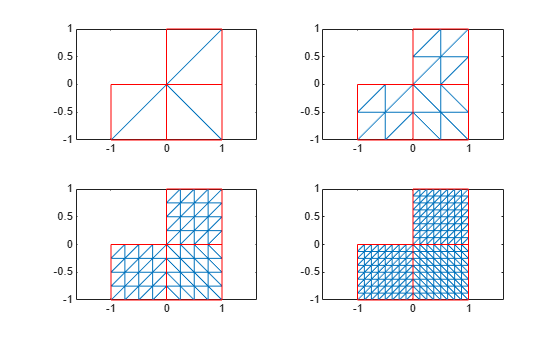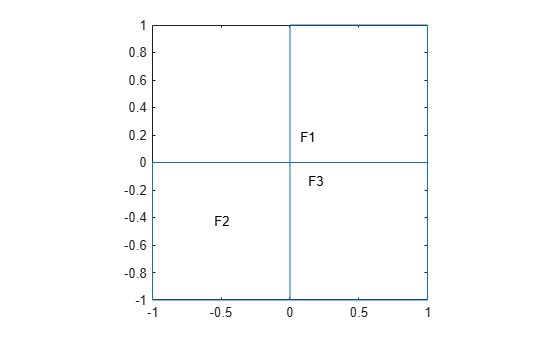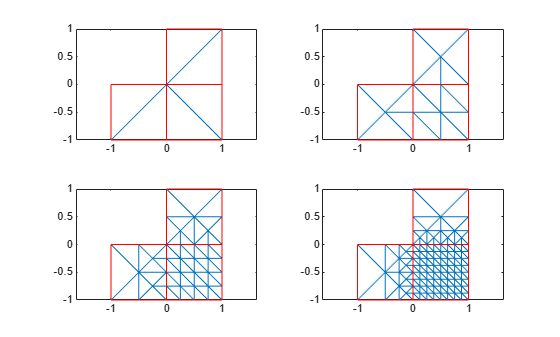# refinemesh

Refine triangular mesh

This page describes the legacy workflow. New features might not be compatible with the legacy workflow. For the corresponding step in the recommended workflow, see `generateMesh`.

## Syntax

``[p1,e1,t1] = refinemesh(g,p,e,t)``
``[p1,e1,t1,u1] = refinemesh(g,p,e,t,u)``
``[___] = refinemesh(___,it)``
``[___] = refinemesh(___,"longest")``

## Description

example

``` NoteThis function does not support quadratic 2-D elements. `[p1,e1,t1] = refinemesh(g,p,e,t)` returns a refined version of the triangular mesh given by the mesh data `p`, `e`, and `t`. For details on the mesh data representation, see Mesh Data as [p,e,t] Triples.```
````[p1,e1,t1,u1] = refinemesh(g,p,e,t,u)` refines the mesh and extends the solution `u` to the new mesh nodes by linear interpolation. The number of rows in `u` must correspond to the number of columns in `p`, and `u1` has as many rows as there are points in `p1`.`refinemesh` interpolates each column of `u` separately.```

example

````[___] = refinemesh(___,it)` uses the input and output arguments from the previous syntaxes and specifies the list `it` of geometric faces or triangles to refine. A scalar or a row vector specifies faces. A column vector specifies triangles.```
````[___] = refinemesh(___,"longest")` uses the longest edge refinement, where the longest edge of each triangle is bisected. By default, `refinemesh` uses the regular refinement, where all triangles are divided into four triangles of the same shape. You also can explicitly specify `"regular"` instead of `"longest"`. If you use a column vector `it` to specify the triangles to refine, then `refinemesh` can refine some triangles outside of the specified set to preserve the triangulation and its quality.```

## Examples

collapse all

Refine the mesh of the L-shaped membrane several times. Plot the initial mesh and refined meshes at each step.

```[p,e,t] = initmesh("lshapeg","Hmax",Inf); subplot(2,2,1) pdemesh(p,e,t) [p,e,t] = refinemesh("lshapeg",p,e,t); subplot(2,2,2) pdemesh(p,e,t) [p,e,t] = refinemesh("lshapeg",p,e,t); subplot(2,2,3) pdemesh(p,e,t) [p,e,t] = refinemesh("lshapeg",p,e,t); subplot(2,2,4) pdemesh(p,e,t)```Refine the mesh for a particular face of the L-shaped membrane.

Plot the L-shaped membrane to identify the face numbers.

`pdegplot("lshapeg","FaceLabels","on")`Create the initial mesh for the entire geometry, then refine the mesh for face 3 several times. Plot the initial mesh and refined meshes at each step.

```[p,e,t] = initmesh("lshapeg","Hmax",Inf); subplot(2,2,1) pdemesh(p,e,t) [p,e,t] = refinemesh("lshapeg",p,e,t,3); subplot(2,2,2) pdemesh(p,e,t) [p,e,t] = refinemesh("lshapeg",p,e,t,3); subplot(2,2,3) pdemesh(p,e,t) [p,e,t] = refinemesh("lshapeg",p,e,t,3); subplot(2,2,4) pdemesh(p,e,t)```## Input Arguments

collapse all

Geometry description, specified as a decomposed geometry matrix, a geometry function, or a handle to the geometry function. For details about a decomposed geometry matrix, see `decsg`. For details about a geometry function, see Parametrized Function for 2-D Geometry Creation.

A geometry function must return the same result for the same input arguments in every function call. Thus, it must not contain functions and expressions designed to return a variety of results, such as random number generators.

Data Types: `double` | `char` | `string` | `function_handle`

Mesh points, specified as a 2-by-`Np` matrix. `Np` is the number of points (nodes) in the mesh. Column `k` of `p` consists of the x-coordinate of point `k` in `p(1,k)` and the y-coordinate of point `k` in `p(2,k)`. For details, see Mesh Data as [p,e,t] Triples.

Mesh edges, specified as a 7-by-`Ne` matrix, where `Ne` is the number of edges in the mesh. An edge is a pair of points in `p` containing a boundary between subdomains, or containing an outer boundary. For details, see Mesh Data as [p,e,t] Triples.

Mesh elements, specified as a 4-by-`Nt` matrix. `Nt` is the number of triangles in the mesh.

The `t(i,k)`, with `i` ranging from 1 through `end - 1`, contain indices to the corner points of element `k`. For details, see Mesh Data as [p,e,t] Triples. The last row, `t(end,k)`, contains the subdomain number of the element.

PDE solution, specified as a vector.

• If the PDE is scalar, meaning that it has only one equation, then `u` is a column vector representing the solution u at each node in the mesh.

• If the PDE is a system of N > 1 equations, then `u` is a column vector with `N*Np` elements, where `Np` is the number of nodes in the mesh. The first `Np` elements of `u` represent the solution of equation 1, the next `Np` elements represent the solution of equation 2, and so on.

Faces or triangles to refine, specified as a positive number or a row or column vector of positive numbers. A scalar or a row vector specifies faces. A column vector specifies triangles.

## Output Arguments

collapse all

Refined mesh points, returned as a 2-by-`Np` matrix. `Np` is the number of points (nodes) in the mesh. Column `k` of `p` consists of the x-coordinate of point `k` in `p(1,k)` and the y-coordinate of point `k` in `p(2,k)`. For details, see Mesh Data as [p,e,t] Triples.

Refined mesh edges, returned as a 7-by-`Ne` matrix, where `Ne` is the number of edges in the mesh. An edge is a pair of points in `p` containing a boundary between subdomains, or containing an outer boundary. For details, see Mesh Data as [p,e,t] Triples.

Refined mesh elements, returned as a 4-by-`Nt` matrix. `Nt` is the number of triangles in the mesh.

The `t(i,k)`, with `i` ranging from 1 through `end-1`, contain indices to the corner points of element `k`. For details, see Mesh Data as [p,e,t] Triples. The last row, `t(end,k)`, contains the subdomain number of the element.

PDE solution, returned as a vector.

• If the PDE is scalar, meaning that it has only one equation, then `u1` is a column vector representing the solution u1 at each node in the mesh.

• If the PDE is a system of N > 1 equations, then `u1` is a column vector with `N*Np` elements, where `Np` is the number of nodes in the mesh. The first `Np` elements of `u1` represent the solution of equation 1, the next `Np` elements represent the solution of equation 2, and so on.

## Algorithms

The refinement algorithm follows these steps:

1. Pick the initial set of triangles to refine.

2. Divide all edges of the selected triangles in half (regular refinement) or divide the longest edge in half (longest edge refinement).

3. Divide the longest edge of any triangle that has a divided edge.

4. Repeat step 3 until no more edges are divided.

5. Introduce new points of all divided edges, and replace all divided entries in `e` by two new entries.

6. Form the new triangles. If all three sides are divided, new triangles are formed by joining the side midpoints. If two sides are divided, the midpoint of the longest edge is joined with the opposing corner and with the other midpoint. If only the longest edge is divided, its midpoint is joined with the opposing corner.

## Version History

Introduced before R2006a